# RD Sharma Solutions for Class 10 Maths Chapter 8 Quadratic Equations MCQs and VSAQs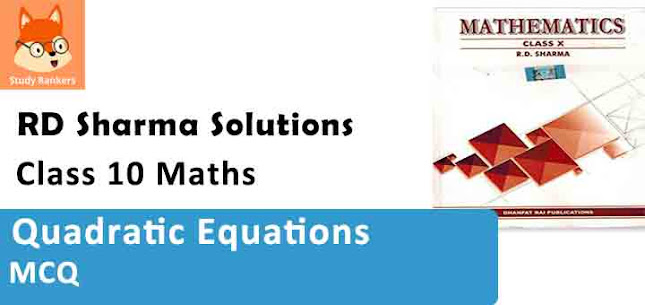Answer each of the following questions either in one word or one sentence or as per requirement of the question :

1. Write the value of k for which the quadratic equation x² – kx + 4 = 0 has equal roots.
Solution

x² – kx + 4 = 0
Here a = 1, b = – k, c = 4
Discriminant (D) = b² – 4ac
= (-k)² – 4×1×4 = k² – 16
The roots are equal
D = 0 ⇒ k² – 16 = 0
⇒ (k + 4) (k – 4) = 0.
Either k + 4 = 0, then k = –4
or k – 4 = 0, then k = 4
k = 4, -4

2. What is the nature of roots of the quadratic equation 4x² – 12x – 9 = 0 ?

Solution

4x² – 12x – 9 = 0
Here a = 4, b = -12, c = – 9
Discriminant (D) = b² – 4ac = (-12)² – 4×4×(-9)
= 144 + 144 = 288
D > 0
Roots are real and distinct.

3. If 1 + √2 is a root of a quadratic equation with rational co-efficients, write its other root.

Solution

The roots of the quadratic equation with rational co-efficients are conjugate
The other root will be 1 – √2.

4. Write the number of real roots of the equation x² + 3|x| + 2 = 0.

Solution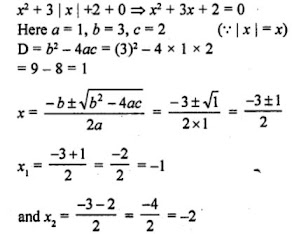∴ Real roots are -1, 2.

5. Write the sum of the real roots of the equation x² + |x| – 6 = 0.
Solution6. Write the set of values of ‘a’ for which the equation x² + ax – 1 = 0, has real roots.
Solution
x² + ax – 1=0
Here a = 1, b = a, c = -1
D = b² – 4ac = (a)² – 4×1×(-1) = a² + 4
Roots are real
D ≥ 0 ⇒ a² + 4 ≥ 0
For all real values of a, the equation has real roots.

7. In there any real value of ‘a’ for which the equation x² + 2x + (a² + 1) = 0 has real roots ?
Solution

x² + 2x + (a² + 1) = 0
D = (-b)² – 4ac = (2)² – 4 x 1 (a² + 1) = 4 – 4a² – 4 = –4a²
For real value of x, D ≥ 0
But – 4a² ≤ 0
So it is not possible
There is no real value of a.

8. Write the value of λ, for which x² + 4x + λ is a perfect square.
Solution

In x² + 4x + λ
a = 1, b = 4, c = λ
x² + 4x + λ will be a perfect square if x² + 4x + λ = 0 has equal roots
D = b² – 4ac = (4)² – 4 x 1 x λ = 16 – 4λ
D = 0
⇒ 16 – 4λ = 0
⇒ 16 = 4A
⇒ λ = 4
Hence λ = 4

9. Write the condition to be satisfied for which equations ax² + 2bx + c = 0 and bx² – 2√ac x + b = 0 have equal roots.
Solution

In ax² + 2bx + c = 0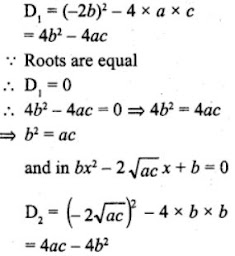Roots are equal.
4ac = 4b2
⇒ b2 = ac
∴ The required condition is b2 = ac.

10. Write the set of values of k for which the quadratic equation has 2x² + kx – 8 = 0 has real roots.
Solution

In 2x² + kx – 8 = 0
D = b²- 4ac = (k)² – 4×2×(-8) = k² + 64
The roots are real
D ≥ 0
k² + 64 ≥ 0
For all real values of k, the equation has real roots.

11. Write a quadratic polynomial, sum of whose zeros is 2√3 and their product is 2.
Solution

Sum of zeros = 2√3
and product of zeros = 2
The required polynomial will be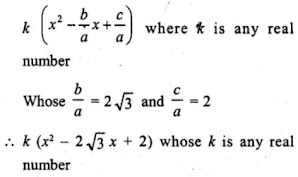12. Show that x = –3 is a solution of x² + 6x + 9 = 0.
Solution

The given equation is x² + 6x + 9 = 0
If x = -3 is its solution then it will satisfy it
L.H.S. = (-3)² + 6 (-3) + 9 = 9 – 18 + 9 = 18 – 18 = 0 = R.H.S.
Hence x = – 3 is its one root (solution)

13. Show that x = –2 is a solution of 3x² + 13x + 14 = 0.
Solution

The given equation is 3x² + 13x + 14 = 0
If x = – 2 is its solution, then it will satisfy it
L.H.S. = 3(-2)² + 13 (- 2) + 14 = 3×4 – 26 + 14
= 12 – 26 + 14 = 26 – 26 = 0 = R.H.S.
Hence x = – 2 is its solution

14. Find the discriminant of the quadratic equation 3√3 x² + 10x + √3 =0.
Solution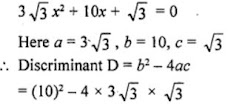= 100 - 12×3 = 100 - 36 = 64

15. If x = −1/2, is a solution of the quadratic equation 3x² + 2kx – 3 = 0, find the value of k.
Solution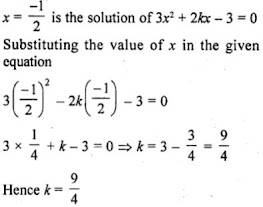Mark the correct alternative in each of the following :

1. If the equation x² + 4x + k = 0 has real and distinct roots, then
(a) k < 4
(b) k > 4
(c) k ≥ 4
(d) k ≤ 4
Solution
(a) In the equation x² + 4x + k = 0
a = 1, b = 4, c = k
D = b² – 4ac = (4)² – 4×1×k = 16 – 4k
Roots are real and distinct
D > 0
⇒ 16 – 4k > 0
⇒ 16 > 4k
⇒ 4 > k
⇒ k < 4

2. If the equation x² – ax + 1 = 0 has two distinct roots, then
(a) |a| = 2
(b) |a| < 2
(c) |a| > 2
(d) None of these
Solution
(c) In the equation x² – ax + 1 = 0
a = 1, b = – a, c = 1
D = b² – 4ac = (-a)² – 4×1×1 = a² – 4
Roots are distinct
D > 0
⇒ a² – 4 > 0
⇒ a² > 4
⇒ a² > (2)²
⇒ |a| > 2

3. If the equation 9x2 + 6kx + 4 = 0, has equal roots, then the roots are both equal to
(a) ± 2/3
(b) ± 3/2
(c) 0
(d) ± 3
Solution
(a)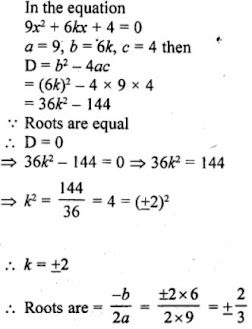4. If ax2 + bx + c = 0 has equal roots, then c =
(a) -b/2a
(b) b/2a
(c) -b²/2a
(d) b²/2a
Solution
(d) In the equation ax2 + bx + c = 0
D = b2 – 4ac
Roots are equal
D = 0
⇒ b2 – 4ac = 0
⇒ 4ac = b2
⇒ c = b2/4a

5. If the equation ax2 + 2x + a = 0 has two distinct roots, if
(a) a = ±1
(b) a = 0
(c) a = 0, 1
(d) a = -1, 0
Solution
(a) In the equation ax2 + 2x + a = 0
D = b2 – 4ac = (2)2 – 4×a× a = 4 – 4a2
Roots are real and equal
D = 0
⇒ 4 – 4a2 = 0
⇒ 4 = 4a2
⇒ 1 = a2
⇒ a2 = 1
⇒ a2 = (±1)2
⇒ a = ±1

6. The positive value of k for which the equation x2 + kx + 64 = 0 and x2 – 8x + k = 0 will both have real roots, is
(a) 4
(b) 8
(c) 12
(d) 16
Solution
(d) In the equation x2 + kx + 64 = 0
a = 1, b = k, c = 64
D = b2 - 4ac = k2 - 4×1×64
⇒ k2 - 256
∵ The roots are real.
∴ D ≥ 0
⇒ k2 - 256 ≥ 0
⇒ k2 ≥ 256
⇒ k2 ≥ (±16)2
⇒ k ≥ 16 ...(1)
Only positive value is taken.
Now in second equation,
x2 - 8x + k = 0
D = (-8)2 - 4×1×k = 64 - 4k
∵ Roots are real
∴ D ≥ 0
⇒ 64 - 4k ≥ 0
⇒ 64 ≥ 4k
⇒ 16 ≥ k ...(2)
From 1 and 2,
16 ≥ k ≥ 16
⇒ k = 16

7.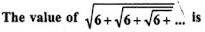(a) 4
(b) 3
(c) -2
(d) 3.5
Solution
(c)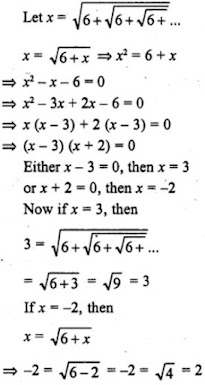which is not possible
∴ x = 3 is correct.

8. If 2 is a root of the equation x2 + bx + 12 = 0 and the equation x2 + bx + q = 0 has equal roots, then q =
(a) 8
(b) – 8
(c) 16
(d) -16
Solution
(c) 16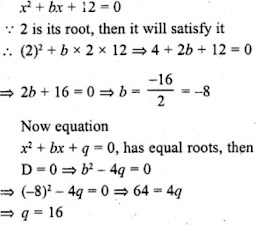9. If the equation (a2 + b2) x2 – 2 (ac + bd) x + c2 + d2 = 0 has equal roots, then

(a) ab = cd
(d) ab = √cd

Solution
(b)
In the equation,

(a2 + b2)x2 - 2(ac + bd)x + (c2 + d2) = 0
D = B- 4AC
= [-2(ac bd)]2 - 4 (a2 + b) (c2 + d2)
= 4[a2c2 + b2d2 + 2abcd] - 4[a2c2 + a2d2 + b2c2 + b2d2]
= 4a2c2 + 4b2d2 + 8abcd - 4a2c2 - 4a2d2 - 4b2c2 - 4b2d2
= 8abcd - 4a2d2 - 4b2c2
= -4[a2d2 + b2c2 - 2abcd]
∵ Roots are equal
∴ D = 0
-4 (ad - bc)2 = 0
⇒ ad - bc = 0

10. If the roots of the equation (a2 + b2) x2 – 2b (a + c) x + (b2 + c2) = 0 are equal, then:

(a) 2b = a + c
(b) b2 = ac
(c) b = 2ac/a+c
(d) b = ac

Solution
(b)
In the equation,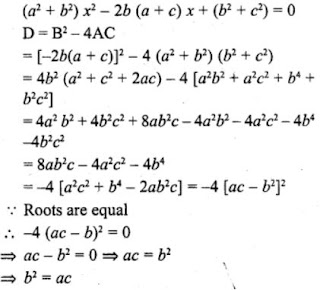11. If the equation x2 – bx + 1 = 0 does not possess real roots, then

(a) -3 < b < 3
(b) -2 < b < 2
(c) b > 2
(d) b < -2

Solution
(b)
In the equation,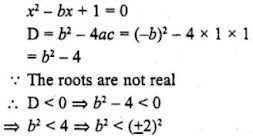∵ b < 2 and b > -2 or -2 < b
∴ -2 < b < 2

12. If x = 1 is a common root of the equations ax2 + ax + 3 = 0 and x2 + x + b = 0, then ab =

(a) 3
(b) 3.5
(c) 6
(d) -3

Solution

(a) In the equation
ax2 + ax + 3 = 0 and x2 + x + b = 0
Substituting the value of x = 1, then in ax2 + ax + 3 = 0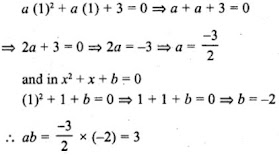13. If p and q are the roots of the equation x2 – px + q + 0, then

(a) p = 1, q = -2
(b) p = 0, q = 1
(c) p = -2, q = 0
(d) p = -2, q = 1

Solution

(a)
p and q are the roots of the equation
x2 - px + q = 0,
Sum of roots = -(-p) = p
Product of roots = q

(a) If p = 1, q = -2, then equation will be
x2 -(s)x + p = 0
⇒ x2 -(1-2)x + 1×(-2) = 0
⇒ x2 + x - 2 = 0

(b) If p = 0, q = 1, then equation will be
x2 - (0 +1)x + 0×1 = 0
⇒ x2 - x + 0 = 0

(c) If p = -2, q = 0, then equation will be
x2 - (-2+0)x +(-2×0)
⇒ x2 + 2x + 0 = 0

(d) p = -2, q = 1, then equation will be
x2 - (-2+1)x + (-2×1) = 0
⇒ x2 + x - 2 = 0
We see that only (a) is correct.
When p = 1, q = -2

14. If a and b can take values 1, 2, 3, 4. Then the number of the equations of the form ax2 + bx + 1 = 0 having real roots is

(a) 10
(b) 7
(c) 6
(d) 12

Solution
(b)
ax2 + bx + 1 = 0
D = b2 – 4a = b2 – 4a
Roots are real.
D ≥ 0
⇒ b2 – 4a ≥ 0
⇒ b2 ≥ 4a
Here, value of b can be 2, 3 or 4
If b = 2, then a can be 1,
If b = 3, then a can be 1, 2
If b = 4, then a can be 1, 2, 3, 4
No. of equation can be 7

15. The number of quadratic equations having real roots and which do not change by squaring their roots is

(a) 4
(b) 3
(c) 2
(d) 1

Solution
(c) There can be two such quad, equations whose roots can be 1 and 0
The square of 1 and 0 remains same
No. of quad equation are 2

16. If (a2 + b2) x2 + 2(ab + bd) x + c2 + d2 = 0 has no real roots, then

(b) ab = cd
(c) ac = bd

Solution
(d)
(a2+b2)x2 + 2(ab+bd)x + c2 + d2 = 0
Here, A = a2 + b2, B = 2 (ab + bd), C = c2 + d2
D = B2 - 4AC = [2(ac + bd)]2 - 4(a2 + b2) (c2 + d2)
= 4[a2c2 + b2d2 + 2abcd] - 4[a2c2 + a2d2+ b2c2 + b2d2]
= 4a2c2 + 4b2d2 + 8abcd - 4a2c2 - 4a2d2 - 4b2c2 - 4b2d2
= -4a2d2 - 4b2c2 + 8abcd
= -4(a2d2 + b2c2 - 2abcd)
∵ Roots are not real.
∴ D < 0
∴ -4 (ad - bc)2 < 0
= (ad - bc)2 < 0

17. If the sum of the roots of the equation x2 – x = λ(2x – 1) is zero, then λ =

(a) -2
(b) 2
(c) –1/2
(d) 1/2

Solution
(c)
x2 – x = λ(2x – 1)
⇒ x2 – x = 2λx – λ
⇒ x2 – x - 2λx + λ = 0
⇒ x2 – (1 + 2λ)x + λ = 0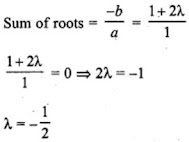18. If x = 1 is a common root of ax2 + ax + 2 = 0 and x2 + x + b = 0 then, ab =

(a) 1
(b) 2
(c) 4
(d) 3

Solution
(b)
ax2 + ax + 2 = 0  ...(i)
x2 + x + b = 0  ...(ii)

x = 1 is common root of equations (i) and (ii)
Then in (i),
a(1)2 + a×1 + 2 = 0
⇒ a + a + 2 = 0
⇒ 2a + 2 = 0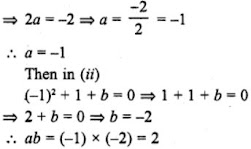19. The value of c for which the equation ax2 + 2bx + c = 0 has equal roots is

(a) b2/a
(b) b2/4a
(c) a2/b
(d) a2/4b

Solution
(a)
ax2 + 2bx + c = 0
D = b- 4ac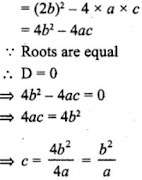20. If x2 + k (4x + k – 1) + 2 = 0 has equal roots, then k =

(a) -2/3, 1
(b) 2/3, -1
(c) 3/2, 1/3
(d) 3/2, -1/3

Solution

(b)
x2 + k (4x + k – 1) + 2 = 0
⇒ x2 + 4kx + k2 – k + 2 = 0
Here, a =1, b = 4k, c =  k2 – k + 2
∵ D = b- 4ac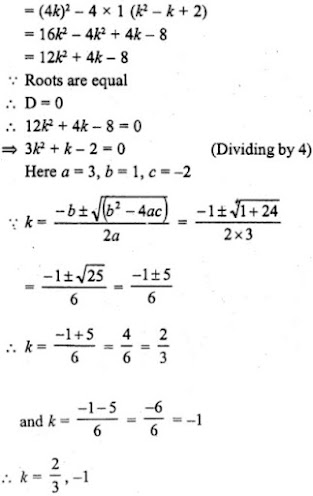21. If the sum and product of the roots of the equation kx2 + 6x + 4k = 0 are equal, then k =

(a) -3/2
(b) 3/2
(c) 2/3
(d) -2/3

Solution

(b)
kx2 + 6x + 4k = 0
Here, a = k, b = 6 and c = 4k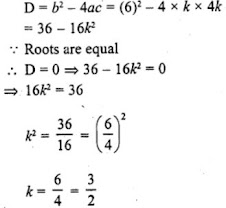22. If sin α and cos α are the roots of the equations ax2 + bx + c = 0, then b2 =

(a) a2 – 2ac
(b) a2 + 2ac
(b) a2 – ac
(d) a2 + ac

Solution
(b)
sin α and cos α are the roots of the equations ax2 + bx + c = 0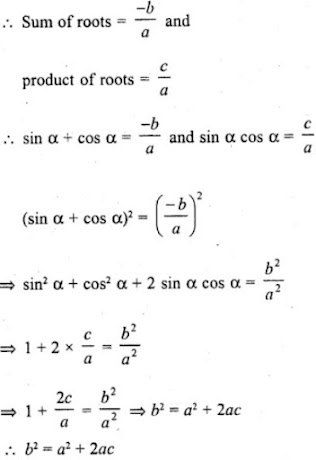23. If 2 is a root of the equation x2 + ax + 12 = 0 and the quadratic equation x2 + ax + q = 0 has equal roots, then q =

(a) 12
(b) 8
(c) 20
(d) 16

Solution
(d)
2 is a root of the equation x2 + ax + 12 = 0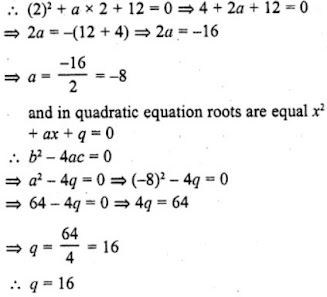24. If the sum of the roots of the equation x2 – (k + 6) x + 2 (2k – 1) = 0 is equal to half of their product, then k =

(a) 6
(b) 7
(c) 1
(d) 5

Solution
x2 – (k + 6) x + 2 (2k – 1) = 0
Here, a = 1, b = – (k + 6), c = 2 (2k – 1)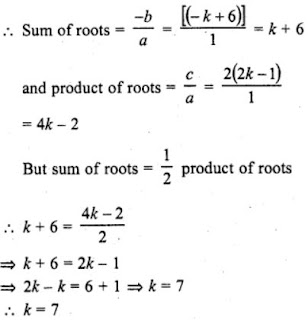25. If a and b are roots of the equation x2 + ax + b = 0, then a + b =

(a) 1
(b) 2
(c) -2
(d) -1

Solution
(d) a and b are the roots of the equation x2 + ax + b = 0
Sum of roots = – a and product of roots = b
Now a + b = –a
and ab = b
⇒ a = 1 …(i)
2a + b = 0
⇒ 2×1 + b = 0
⇒ b = -2
Now, a + b = 1 – 2 = -1

26. A quadratic equation whose one root is 2 and the sum of whose roots is zero, is

(a) x2 + 4 = 0
(b) x2 – 4 = 0
(c) 4x2 – 1 = 0
(d) x2 – 2 = 0

Solution
(b) Sum of roots of a quad, equation = 0
One root = 2
Second root = 0 – 2 = – 2
and product of roots = 2×(-2) = – 4
Equation will be
x2 + (sum of roots) x + product of roots = 0
x2 + 0x + (-4) = 0
⇒ x2 – 4 = 0

27. If one root of the equation ax2 + bx + c = 0 is three times the other, then b2 : ac =

(a) 3 : 1
(b) 3 : 16
(c) 16 : 3
(d) 16 : 1

Solution
(c)
Equation is ax2 + bx + c = 0
Let first root = ɑ then,
Second rot = 3ɑ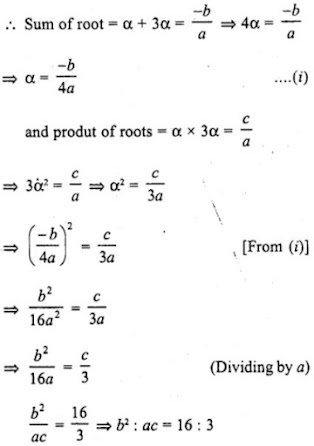28. If one root of the equation 2x2 + kx + 4 = 0 is 2, then the other root is

(a) 6
(b) -6
(c) -1
(d) 1

Solution
(d) The given quadratic equation 2x2 + kx + 4 = 0
One root is 2
Product of roots = c/a = 4/2 = 2
Second root = 2/2 = 1

29. If one root of the equation x2 + ax + 3 = 0 is 1, then its other root is
(a) 3
(b) -3
(c) 2
(d) -2

Solution
(a) The quad, equation is x2 + ax + 3 = 0
One root =1
and product of roots = c/a = 3/1 = 3
Second root = 3/1 = 3

30. If one root of the equation 4x2 – 2x + (λ – 4) = 0 be the reciprocal of the other, then λ =
(a) 8
(b) -8
(c) 4
(d) -4

Solution
(a)
The quadratic equation is 4x2 – 2x + (λ – 4) = 0
Let first root be ɑ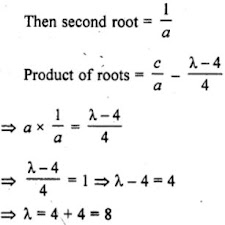31. If y = 1 is a common root of the equations ay2 + ay + 3 = 0 and y2 + y + b = 0, then ab equals

(a) 3
(b) – 1/2
(c) 6
(d) -3

Solution
(a)
y = 1
ay2 + ay + 3 = 0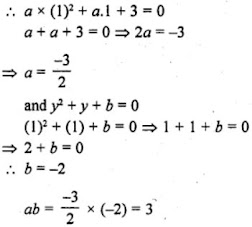32. The values of k for which the quadratic equation 16x2 + 4kx + 9 = 0 has real and equal roots are

(a) 6, –1/6
(b) 36, -36
(c) 6, -6
(d) 3/4 , –3/4

Solution
(c) 16x2 + 4kx + 9 = 0
Here a = 16, b = 4k, c = 9
Now D = b2 – 4ac = (4k)2 – 4×16×9 = 16k2 – 576
Roots are real and equal
D = 0 or b2 – 4ac = 0
⇒ 16k2 – 576 = 0
⇒ k2 – 36 = 0
⇒ k2 = 36 = (± 6)2
k = ± 6
k = 6, -6